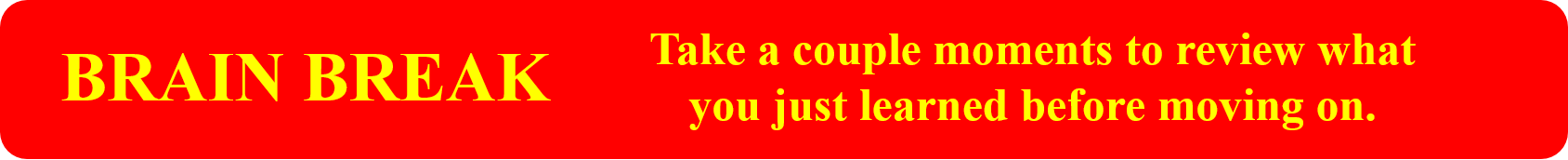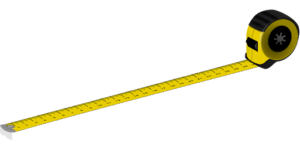Decimals

# 14 Convert Decimals to Fractions

Chad has decided he wants to work with money in fraction form and not in decimal form. Why, you ask? I don’t know. Work with me here, though, because it’s been hard to get Chad into the decimal story. Maybe he just wants his students to know a little more math. No harm there. What we’re looking at here is the following:

$\LARGE0.5\text{ to }\dfrac{1}{2}$

It’s important to know how to do this in order to work with different number forms in the workplace. For instance, David, a student in Chad’s class, is a machinist. Machinists produce metal parts with a precision of up to one one-thousandth of a inch. If you are continuously working in decimals, you might forget how to work with fractions, so David wants to know how to change those decimals into fractions. The best way to illustrate how to do this is simply with an example.

Example

We’ll start with an easy decimal like 0.25 and work towards changing this into a fraction.

Step 1: Put the question into fraction form such that the decimal is over 1.

$\LARGE\dfrac{0.25}{1}$

Step 2: Take both the numerator and the denominator and multiply each by 10 for every digit to the right of the decimal point. In this case, we have 2 digits to the right. Therefore, we multiply each by 100 (10 × 10).Step 3: Reduce the fraction to its lowest terms, and we end up with the final answer.Example

Change the decimal 0.729 into a fraction.

Step 1: Put the equation into fraction form such that the decimal is over 1.

$\LARGE\dfrac{0.729}{1}$

Step 2: Take both the numerator and the denominator and multiply each by 10 for every digit to the right of the decimal point. In this case, we have 3 digits to the right. Therefore, we multiply each by 1000 (10 × 10 × 10).Step 3: Reduce the fraction to get our final answer.

This is a tough one to reduce. My suggestion would be to do this in steps. Start with small numbers like 2 and 3. Does the number 2 go into both the numerator and the denominator? The answer would be no. How about 3? Also no. Continue this process until you find a number that works.

It is possible that you will not find a number and we are already in lowest terms. This is the case here. Our final answer is simply:

$\LARGE\dfrac{729}{1000}$

# Practice Questions

Try a couple practice questions and check the video answers.

Change the following decimals into fractions. Put your answer in lowest terms.

Question 1

$\LARGE0.362$

Question 2

$\LARGE0.963$Now it’s time to move to a situation we might find on a job site. Have you ever been on a job site, and your boss asked you to cut a piece of pipe, or maybe a piece of wood? What was the measurement? Maybe it was something like 8 ⅜ inches. Maybe it was 2 feet, 2 ¼ inches. Those are pretty standard.

But what if you were asked to cut a piece of material 2.9384 feet? For David, the machinist, this might be exactly the type of measurement that he would get while creating metal products. But what would you do in some of the other trades?

This is the reason we need to be able to change decimals into fractions. The whole number in this example stays the same, so we don’t really need to deal with that. What we need to work with is the 0.9384.

The goal is to change 2.9384 into feet, inches, and fractions of an inch. We’ll go through an example to show you how that is done.

Example

Step 1: First, note that the number we are dealing with is in feet. We have 2.9384 feet. The 2 whole feet will not require any changing and is good the way it is. What we have to do first is change 0.9384 into inches before moving onto fractions of an inch. Start with how many inches there are in a foot.

$\LARGE1\text{ foot}=12\text{ inches}$

Take the decimal of a foot and multiply it by 12.

$\LARGE0.9384\times12=11.2608\text{ inches}$

Step 2: We are left with 11 inches and decimals of an inch. The 11 inches is good the way it is, but we need to change the decimal of an inch into fractions of an inch. The question becomes, which fraction of an inch should we change it into?

This is up to you. This could be fourths, eighths, sixteenths, or thirty-seconds. The most common way would be to change the decimal of an inch into sixteenths. This is done by multiplying the decimal of an inch by the number in the denominator of whichever fraction you are working towards. For our example, we are looking to convert the decimal into sixteenths, so we multiply it by 16.

$\LARGE0.2608\times16=4.1728$

When we are looking at 4.1728, what we are actually looking at is:

$\LARGE\dfrac{4.1728}{16}$

If we had taken the 0.2608 inches and multiplied it by 8, our answer would be in eighths of an inch. If we had multiplied by 4, our answer would be in fourths (or quarters) of an inch.

Step 3: Round the answer to the nearest fraction of an inch, and then reduce the fraction if necessary.

$\LARGE\dfrac{4.1728}{16}\rightarrow\dfrac{4}{16}\rightarrow\dfrac{1}{4}$

Our final answer then becomes:Example

Change the following feet and decimals of a foot into feet, inches, and sixteenths of an inch.

$\LARGE7.6939\text{ feet}$

Step 1: Change the decimal of a foot into inches.

$\LARGE0.6939\times12=8.3268\text{ inches}$

Step 2: We are left with 8 inches and decimals of an inch. The 8 inches is good the way it is, but we need to change the decimal of an inch into fractions of an inch. We need to change the decimal of an inch into sixteenths, so multiply it by 16.

$\LARGE0.3268\times16=5.2288$

Step 3: Round the answer to the nearest fraction of an inch, and then reduce the fraction if necessary.

$\LARGE\dfrac{5.2288}{16}\rightarrow\dfrac{5}{16}$

Our final answer becomes:# Practice Questions

Change the following feet and decimals of a foot into feet, inches, and sixteenths of an inch. Put your answer in lowest terms and check out the videos answers when you are done.

Question 1

$\LARGE9.1234\text{ feet}$

Question 2

$\LARGE0.058\text{ feet}$